Chapter 7: Factoring

# 7.9 Age Word Problems

One application of linear equations is what are termed age problems. When solving age problems, generally the age of two different people (or objects) both now and in the future (or past) are compared. The objective of these problems is usually to find each subject’s current age. Since there can be a lot of information in these problems, a chart can be used to help organize and solve. An example of such a table is below.

Person or Object Current Age Age Change

Example 7.9.1

Joey is 20 years younger than Becky. In two years, Becky will be twice as old as Joey. Fill in the age problem chart, but do not solve.

• The first sentence tells us that Joey is 20 years younger than Becky (this is the current age)
• The second sentence tells us two things:
1. The age change for both Joey and Becky is plus two years
2. In two years, Becky will be twice the age of Joey in two years
Person or Object Current Age Age Change (+2)
Joey (J) B − 20 B − 20 + 2
B − 18
Becky (B) B B = 2

Using this last statement gives us the equation to solve:

B + 2  =  2 ( B − 18)

Example 7.9.2

Carmen is 12 years older than David. Five years ago, the sum of their ages was 28. How old are they now?

• The first sentence tells us that Carmen is 12 years older than David (this is the current age)
• The second sentence tells us the age change for both Carmen and David is five years ago (−5)

Filling in the chart gives us:

Person or Object Current Age Age Change (−5)
Carmen (C) D + 12 D + 12 − 5
D + 7
David (D) D D − 5

The last statement gives us the equation to solve:

Five years ago, the sum of their ages was 28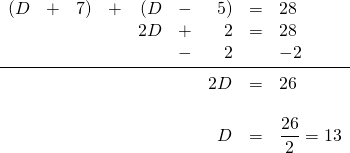Therefore, Carmen is David’s age (13) + 12 years = 25 years old.

Example 7.9.3

The sum of the ages of Nicole and Kristin is 32. In two years, Nicole will be three times as old as Kristin. How old are they now?

• The first sentence tells us that the sum of the ages of Nicole (N) and Kristin (K) is 32. So N + K = 32, which means that N = 32 − K or
K = 32 − N (we will use these equations to eliminate one variable in our final equation)
• The second sentence tells us that the age change for both Nicole and Kristen is in two years (+2)

Filling in the chart gives us:

Person or Object Current Age Age Change (+2)
Nicole (N) N N + 2
Kristin (K) 32 − N (32 − N) + 2
34 − N

The last statement gives us the equation to solve:

In two years, Nicole will be three times as old as Kristin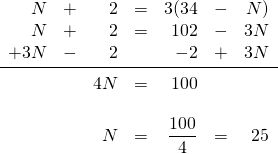If Nicole is 25 years old, then Kristin is 32 − 25 = 7 years old.

Example 7.9.4

Louise is 26 years old. Her daughter Carmen is 4 years old. In how many years will Louise be double her daughter’s age?

• The first sentence tells us that Louise is 26 years old and her daughter is 4 years old
• The second line tells us that the age change for both Carmen and Louise is to be calculated (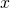)

Filling in the chart gives us:

Person or Object Current Age Age Change
Louise (L)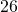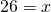Daughter (D)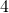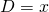The last statement gives us the equation to solve:

In how many years will Louise be double her daughter’s age?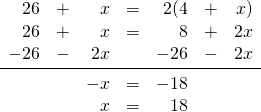In 18 years, Louise will be twice the age of her daughter.

# Questions

For Questions 1 to 8, write the equation(s) that define the relationship.

1. Rick is 10 years older than his brother Jeff. In 4 years, Rick will be twice as old as Jeff.
2. A father is 4 times as old as his son. In 20 years, the father will be twice as old as his son.
3. Pat is 20 years older than his son James. In two years, Pat will be twice as old as James.
4. Diane is 23 years older than her daughter Amy. In 6 years, Diane will be twice as old as Amy.
5. Fred is 4 years older than Barney. Five years ago, the sum of their ages was 48.
6. John is four times as old as Martha. Five years ago, the sum of their ages was 50.
7. Tim is 5 years older than JoAnn. Six years from now, the sum of their ages will be 79.
8. Jack is twice as old as Lacy. In three years, the sum of their ages will be 54.

Solve Questions 9 to 20.

1. The sum of the ages of John and Mary is 32. Four years ago, John was twice as old as Mary.
2. The sum of the ages of a father and son is 56. Four years ago, the father was 3 times as old as the son.
3. The sum of the ages of a wood plaque and a bronze plaque is 20 years. Four years ago, the bronze plaque was one-half the age of the wood plaque.
4. A man is 36 years old and his daughter is 3. In how many years will the man be 4 times as old as his daughter?
5. Bob’s age is twice that of Barry’s. Five years ago, Bob was three times older than Barry. Find the age of both.
6. A pitcher is 30 years old, and a vase is 22 years old. How many years ago was the pitcher twice as old as the vase?
7. Marge is twice as old as Consuelo. The sum of their ages seven years ago was 13. How old are they now?
8. The sum of Jason and Mandy’s ages is 35. Ten years ago, Jason was double Mandy’s age. How old are they now?
9. A silver coin is 28 years older than a bronze coin. In 6 years, the silver coin will be twice as old as the bronze coin. Find the present age of each coin.
10. The sum of Clyde and Wendy’s ages is 64. In four years, Wendy will be three times as old as Clyde. How old are they now?
11. A sofa is 12 years old and a table is 36 years old. In how many years will the table be twice as old as the sofa?
12. A father is three times as old as his son, and his daughter is 3 years younger than his son. If the sum of all three ages 3 years ago was 63 years, find the present age of the father.